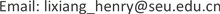1. 引言

2. 数字可编程超表面无线通信系统模型

2.1. 数字可编码超表面的结构及载波调制原理

R n ( t ) = Γ n ( t ) ⋅ I n ( t ) (1)

2.2. 不同数字调制方案下发射机对超表面数字驱动电压序列的设计

Γ ( t ) = Γ m h ( t ) , 0 ≤ t ≤ T , Γ m ∈ A (2)

Γ m ∈ A = { e j π 4 , e j 3 π 4 , e j 5 π 4 , e j 7 π 4 } , | A | = 4 , m = 0 , 1 , 2 , 3 (3)

Γ ( t ) = Γ m h ( t ) , 0 ≤ t ≤ T (4)

Γ m = e j 2 π f m t , f m ∈ A = { f 0 , ⋯ , f M − 1 } (5)

Γ ( t ) = ∑ p P − 1 Γ p g ( t − p τ ) , 0 ≤ t ≤ T , Γ p ∈ A (6)

g ( t ) = 1 , 0 ≤ t ≤ τ (7)

Γ ( t ) = ∑ k = − ∞ + ∞ a k e j k 2 π f 0 t , 0 ≤ t ≤ T (8)

g ( t ) = ∑ k = − ∞ + ∞ c k e j k 2 π f 0 t , 0 ≤ t ≤ τ (9)

c k = 1 P S a ( k π P ) e − j k π P (10)

Γ ( t ) = ∑ p = 0 P − 1 Γ p g ( t − p τ ) = ∑ k = − ∞ + ∞ c k ( ∑ p = 0 P − 1 Γ p g − j 2 k p π P ) e j k 2 π f 0 t , 0 ≤ t ≤ T (11)

a k = A k ∑ p = 0 P − 1 Γ p e − j 2 k p π P (12)

2.3. 未来超表面无线通信系统可能的结构和应用方式

3. 数字可编程超表面无线通信系统的硬件实现

3.1. 系统架构和参数

List of devices and hardwar

NI PXIe-1082机箱用于装载NI系列硬件，并提供硬件连接使其数据互通
NI PXIe-8135控制器作为发射端上位机，将用户数据(图片)翻译成比特流，再映射并量化为相位符号
NI FPGA 7966R将量化后的相位符号转化为实际的数字驱动电压
NI DA 5781R对数字驱动电压进行插值调整等操作
KeysightE8267D信号源用于发射端产生单音载波信号，并通过喇叭天线辐射向超表面
NI USRP-RIO 2943R用于接收端数据接收、检测和判决
DELL笔记本作为接收端上位机，恢复出原始用户数据(图片)并显示相关参数

List of system parameter

FSK频点(比特“0”和“1”)3.8 GHz ± 78.125 kHz

FFT点数512

3.2. 收发端关键代码及操作

LabVIEWFPGA是美国国家仪器公司推出的一款图形化界面FPGA开发语言，具有易开发、可释性强等优点，本文采用该语言进行数字可编程超表面无线通信系统的实现。下面给出该系统收发端关键的关键操作和模块代码。

3.3. 测试结果及讨论

4. 结论